DGtal  1.3.beta
Embedding n-dimensional DEC structures into m-dimensional space

Author(s) of this documentation:
Pierre Gueth

# Introduction

The DEC package allows the embedding of a discrete manifold contained in an higher dimension Euclidean space. For example, one could use the DEC package to represent a curved surface embedded in a three dimensional Euclidean space. Throughout this documentation $$n$$ stands for in embedded manifold dimension, $$m$$ stands for this ambient space dimension and $$k$$ is used to characterize the dimension of cells inside the DEC structure.

$0 \le k \le n \le m$

Only lower dimensions k-cells can be inserted into a DEC structure ( $$k \le n$$). Those embedded and ambient dimensions are enforced as the first and second template parameters of DiscreteExteriorCalculus. Dual and primal operators are defined with respect the the embedding dimension.

# DEC factory

DiscreteExteriorCalculusFactory is a helper class to easily create DEC structures from digital sets or combinatorial structures such as digital surfaces (DigitalSurface).

As usual, one can fill the DEC structure manually using DiscreteExteriorCalculus.insertSCell. Every k-cells should then be inserted manually with their correct primal and dual sizes. Primal and dual sizes default to 1 when not specified. On the other hand, one could create automatically a DEC structure from a range of n-cells using DiscreteExteriorCalculusFactory::createFromNSCells. Incident lower dimension cells are then inserted and weighted automatically. The first template parameter of DiscreteExteriorCalculusFactory::createFromNSCells represents the embedded dimension of the generated manifold.

Note
By definition 0-cell must have a primal size equals to 1 and n-cell must have dual size equals to 1.

When the ambient space has the same dimension as the embedded manifold, one could use DiscreteExteriorCalculusFactory::createFromDigitalSet. Similarly to DiscreteExteriorCalculusFactory::createFromNSCells, incident lower dimension cells are automatically inserted with proper primal and dual sizes. Each point of the set is inserted in the DEC structure as a primal n-cell.

Note
DiscreteExteriorCalculusFactory::createFromNSCells is compatible with iterators over DigitalSurface.

To define a DEC factory, one must pass the linear algebra backend as the first template parameter. Here is a snippet that define a factory using EigenLinearAlgebraBackend.

typedef DiscreteExteriorCalculusFactory<EigenLinearAlgebraBackend> CalculusFactory;

# Border definition

When using a DEC factory to generate DEC structures, one could specify if borders are inserted in the generated structure using the second parameter add_border. Cell belonging to the border are (n-1)-cells incident with to one n-cell, along with their lower incident k-cells counterparts. When passing add_border=false to DEC factory static functions, cells belonging to the border are not inserted.

Note
Choosing to add borders or not changes boundary condition of the DEC problems generated with the structure. add_border=true enforces Neumann boundary condition on primal operators. add_border=false enforces Dirichlet boudary condition on primal operators. See 1D Poisson resolution for an example.
add_border boundary condition of primal operators boundary condition of dual operators
true Neumann $$\nabla f(x) \cdot n(x)=0~\forall x \in \partial M$$ Dirichlet $$f(x)=0~\forall x \in \partial M$$
false Dirichlet $$f(x)=0~\forall x \in \partial M$$ Neumann $$\nabla f(x) \cdot n(x)=0~\forall x \in \partial M$$

# 1D examples

In this example, we show how to embed linear structure into different ambient spaces. See testEmbedding.cpp for details. First, let's start be defining types of DEC structures used in this example.

typedef DiscreteExteriorCalculus<1, 1, EigenLinearAlgebraBackend> Calculus1D;
typedef DiscreteExteriorCalculus<1, 2, EigenLinearAlgebraBackend> Calculus2D;
typedef DiscreteExteriorCalculus<1, 3, EigenLinearAlgebraBackend> Calculus3D;

Calculus1D represents a 1D discrete manifold embedded in 1D Euclidean space, Calculus2D represents a 1D discrete manifold embedded in 2D Euclidean space and Calculus3D represents a 1D discrete manifold embedded in 3D Euclidean space.

After defining and filling the input forward traversal containers (std::set, std::list, std::vector, ...), DEC structures are generated by calling DiscreteExteriorCalculusFactory::createFromNSCells.

typedef std::set<Calculus1D::SCell> SCells1D;
SCells1D ncells_1d_factory;
const Calculus1D calculus_1d_factory = CalculusFactory::createFromNSCells<1>(ncells_1d_factory.begin(), ncells_1d_factory.end(), true);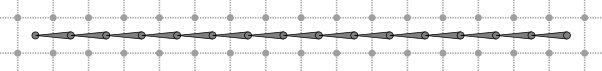1D discrete manifold embedded in 1D ambient space.
typedef std::list<Calculus2D::SCell> SCells2D;
SCells2D ncells_2d_factory;
const Calculus2D calculus_2d_factory = CalculusFactory::createFromNSCells<1>(ncells_2d_factory.begin(), ncells_2d_factory.end(), true);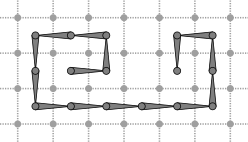1D discrete manifold embedded in 2D ambient space.
typedef std::vector<Calculus3D::SCell> SCells3D;
SCells3D ncells_3d_factory;
const Calculus3D calculus_3d_factory = CalculusFactory::createFromNSCells<1>(ncells_3d_factory.begin(), ncells_3d_factory.end(), true);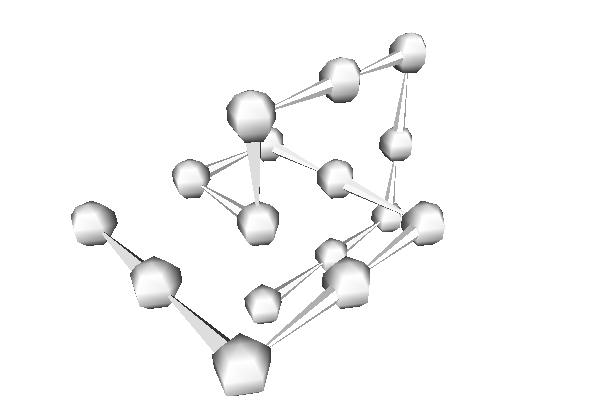1D discrete manifold embedded in 3D ambient space.

Operators generated from these structures are all identical.

# 2D examples

In this example, we show how to discrete curved surface into different ambient spaces. See testEmbedding.cpp for details. First, let's start be defining types of DEC structures used in this example.

typedef DiscreteExteriorCalculus<2, 2, EigenLinearAlgebraBackend> Calculus2D;
typedef DiscreteExteriorCalculus<2, 3, EigenLinearAlgebraBackend> Calculus3D;

Calculus2D represents a 2D discrete manifold embedded in 2D Euclidean space and Calculus3D represents a 2D discrete manifold embedded in 3D Euclidean space.

After defining and filling the input forward traversal containers (std::set, std::list, std::vector, ...), DEC structures are generated by calling DiscreteExteriorCalculusFactory::createFromNSCells.

typedef std::list<Calculus2D::SCell> SCells2D;
SCells2D ncells_2d_factory;
const Calculus2D calculus_2d_factory_weighed = CalculusFactory::createFromNSCells<2>(ncells_2d_factory.begin(), ncells_2d_factory.end(), true);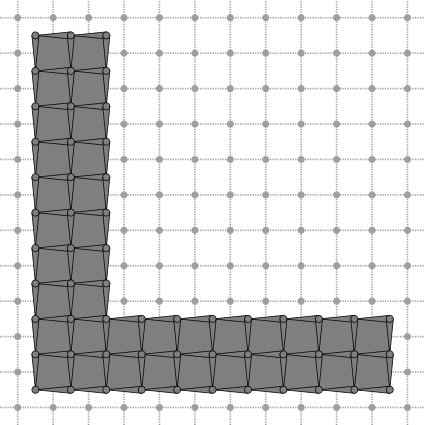2D discrete manifold embedded in 2D ambient space with border.
typedef std::list<Calculus3D::SCell> SCells3D;
SCells3D ncells_3d_factory;
const Calculus3D calculus_3d_factory_weighed = CalculusFactory::createFromNSCells<2>(ncells_3d_factory.begin(), ncells_3d_factory.end(), true);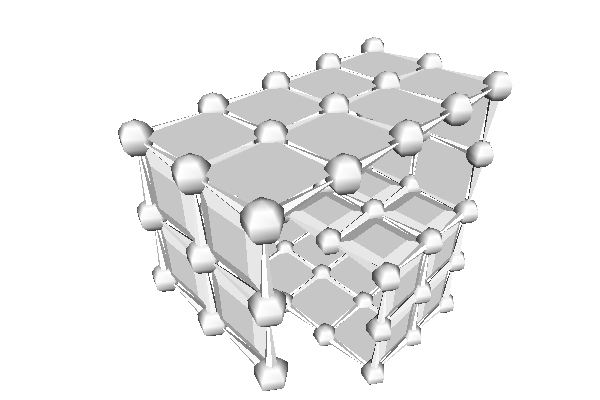2D discrete manifold embedded in 3D ambient space with border.

Borderless DEC structures are generated by calling DiscreteExteriorCalculusFactory::createFromNSCells with third parameter equals to false.

const Calculus2D calculus_2d_factory_no_border = CalculusFactory::createFromNSCells<2>(ncells_2d_factory.begin(), ncells_2d_factory.end(), false);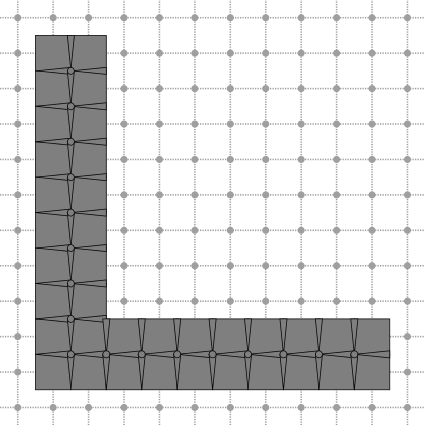2D discrete manifold embedded in 2D ambient space without border.
const Calculus3D calculus_3d_factory_no_border = CalculusFactory::createFromNSCells<2>(ncells_3d_factory.begin(), ncells_3d_factory.end(), false);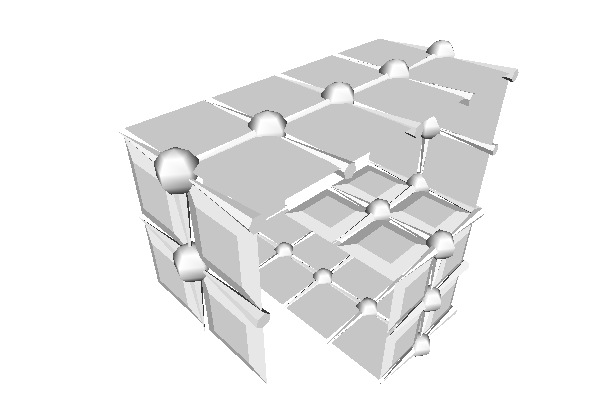2D discrete manifold embedded in 3D ambient space without border.

Embedding structure in higher dimension ambient space does not affect generated operators.

# Digital surface examples

In this example, we show how to import a DigitalSurface into a DEC structure. See exampleDECSurface.cpp for details. First, let's define the input set whose boundary will be used to define the digital surface.

DGtal::Z3i::DigitalSet input_set(input_domain);
input_set.insert( DGtal::Z3i::Point(0,0,0) );
input_set.insert( DGtal::Z3i::Point(1,0,0) );
input_set.insert( DGtal::Z3i::Point(1,1,0) );
input_set.insert( DGtal::Z3i::Point(0,1,0) );
input_set.insert( DGtal::Z3i::Point(0,0,1) );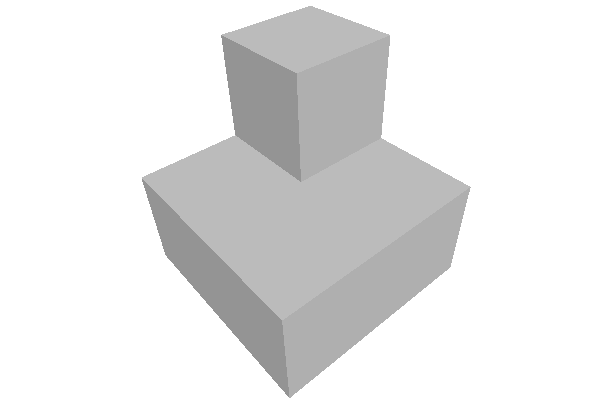Input digital set.

Let's then define the digital surface as the boundary of the input set, oriented positive inward.

const DigitalSurfaceContainer digital_surface_container(kspace, input_set, surfel_adjacency, cell_bel);
const DigitalSurface digital_surface(digital_surface_container);

The DEC structure is created by calling DiscreteExteriorCalculusFactory::createFromNSCells with iterators DigitalSurface.begin() and DigitalSurface.end(). Incident lower cells are added automatically as well as Hodge weights.

const Calculus calculus = CalculusFactory::createFromNSCells<2>(digital_surface.begin(), digital_surface.end());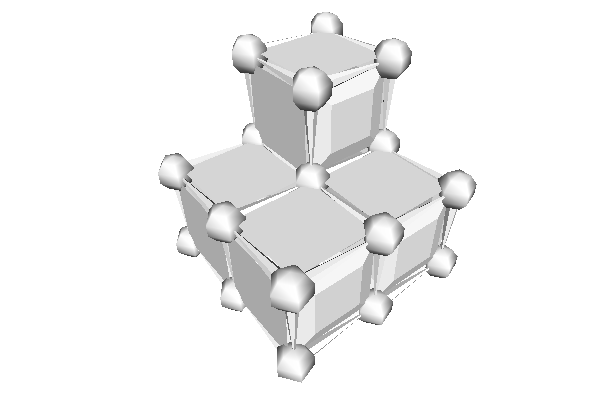DEC structure from boundary digital set.
DGtal::DiscreteExteriorCalculus
Aim: DiscreteExteriorCalculus represents a calculus in the dec package. This is the main structure in...
Definition: DiscreteExteriorCalculus.h:97
DGtal::DigitalSurface
Aim: Represents a set of n-1-cells in a nD space, together with adjacency relation between these cell...
Definition: DigitalSurface.h:139
Aim: Represent adjacencies between surfel elements, telling if it follows an interior to exterior ord...
DGtal::SignedKhalimskyCell
Represents a signed cell in a cellular grid space by its Khalimsky coordinates and a boolean value.
Definition: KhalimskySpaceND.h:208
DGtal::LightImplicitDigitalSurface
Aim: A model of CDigitalSurfaceContainer which defines the digital surface as the boundary of an impl...
Definition: LightImplicitDigitalSurface.h:115
DGtal::Surfaces::findABel
static SCell findABel(const KSpace &K, const PointPredicate &pp, unsigned int nbtries=1000)
DGtal::DiscreteExteriorCalculusFactory
Aim: This class provides static members to create DEC structures from various other DGtal structures.
Definition: DiscreteExteriorCalculus.h:70
DGtal::PointVector< dim, Integer >
DGtal::DigitalSetByAssociativeContainer
Aim: A wrapper class around a STL associative container for storing sets of digital points within som...
Definition: DigitalSetByAssociativeContainer.h:89